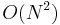Constant optimization

Constant optimization is sometimes required in order to score full points on a contest problem with a "tight" time limit. If there are up to 100000 lines of input and you write a program whose algorithm is$O(N \log N)$, your program might be too slow; on the other hand, if your algorithm is$O(N^2)$, then it is almost certainly too slow, and trying to optimize it to$O(N \log N)$ should be undertaken before optimizing the constant factor.